The password to open the file is -  teachooisbest

## A person while waving his hand very fast in front of his eyes, observes that his fingers appear blurred. What could be the reason for it?

The image of an object seen by our eye remains for about 1/16 th of a second on the retina.

So, If a still image is flashed at a rate faster than 16 times per second, then the image appears to be moving.

In this case, the hand moves very fast (much more than 16 times per second).

Thus, it appears blurred.

## The distance between the object and its image formed by a plane mirror appears to be 24 cm. What is the distance between the mirror and the object?

In a Plane mirror,

The image formed is at the same distance behind the mirror as the object is in front of it.

Hence,

Distance between object and mirror = Distance between mirror and image = d

Hence,

Distance between object and image = d + d

= 2d

Given,

Distance between object and image = 24cm

Therefore,

2d = 24 cm

d = 24/2

d = 12 cm

Thus, Distance between object and mirror is 12 cm

## Eyes of the nocturnal birds have large cornea and a large pupil. How does this structure help them?

The birds, like owl, which are widely active at night are known as nocturnal birds.

These birds have large cornea and large pupils so that they can collect large amounts of light, which would help them to see better at night.

## There is a mistake in, each of the following ray diagrams given as Fig. (a), (b), and (c). Make the necessary correction(s).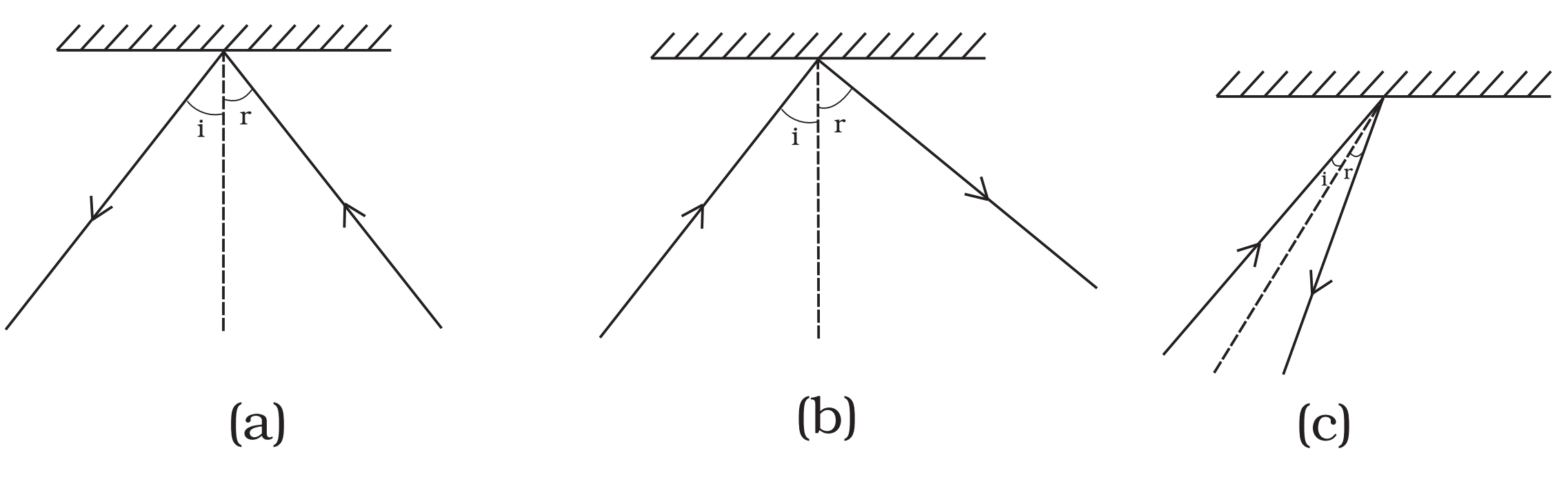(a) The direction of incident wave should be towards the mirror and the direction of reflected wave should be away from the mirror.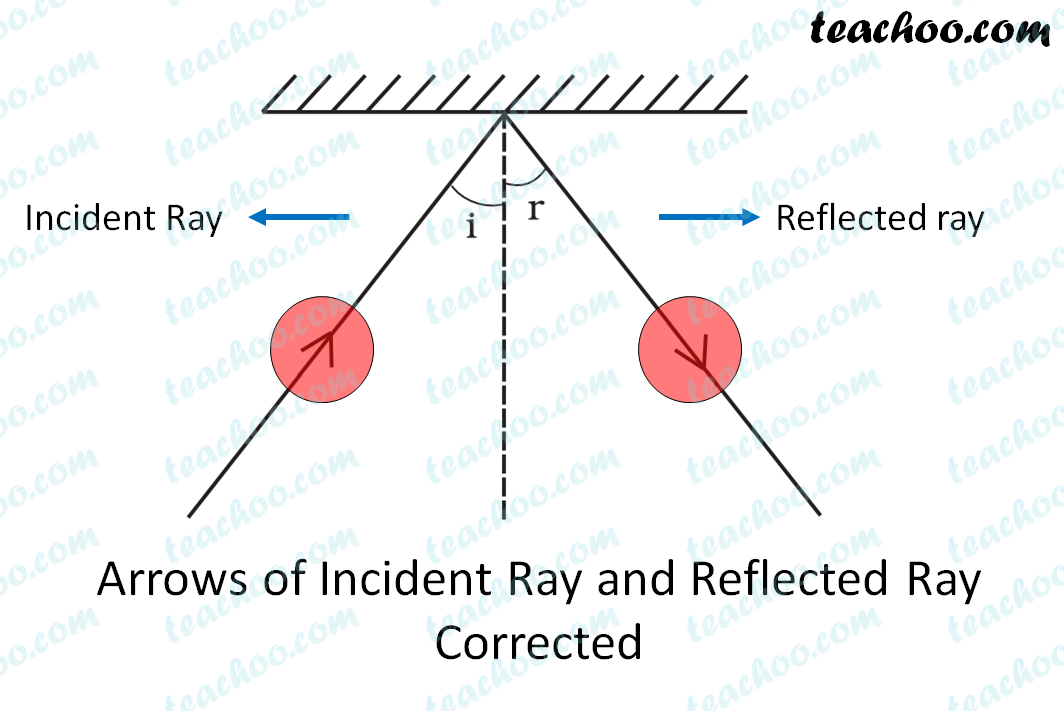(b) The angle of reflection should be equal to the angle of incidence.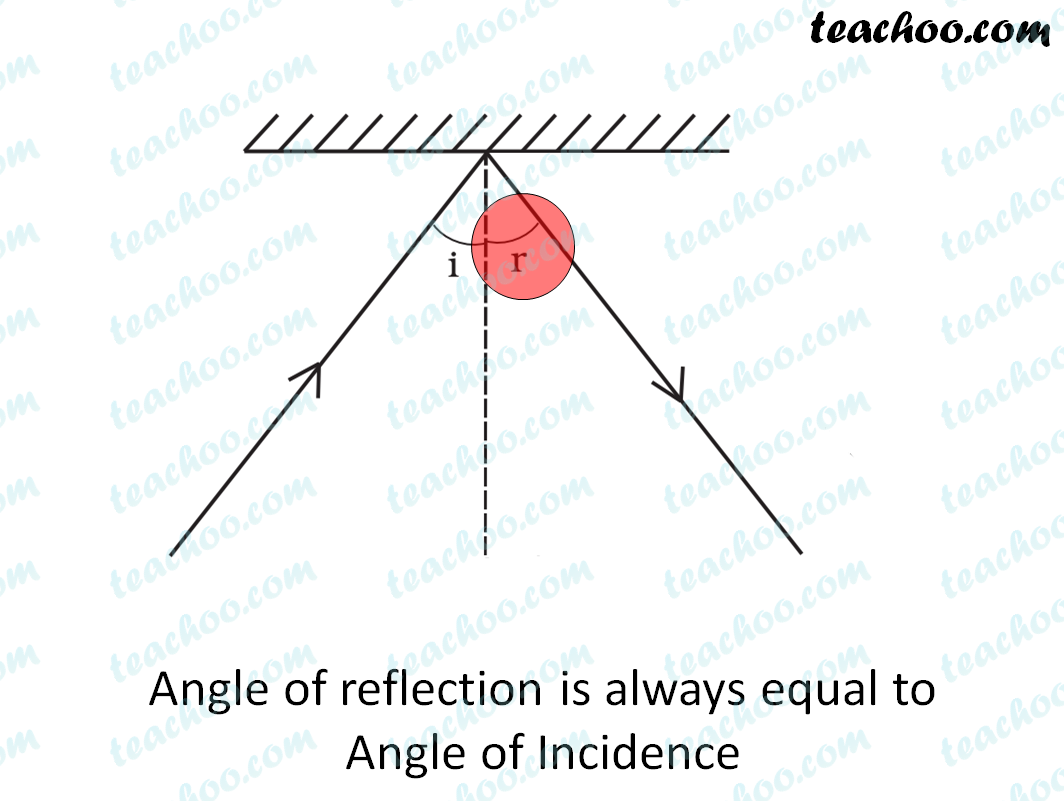(c) The normal should be perpendicular to the mirror.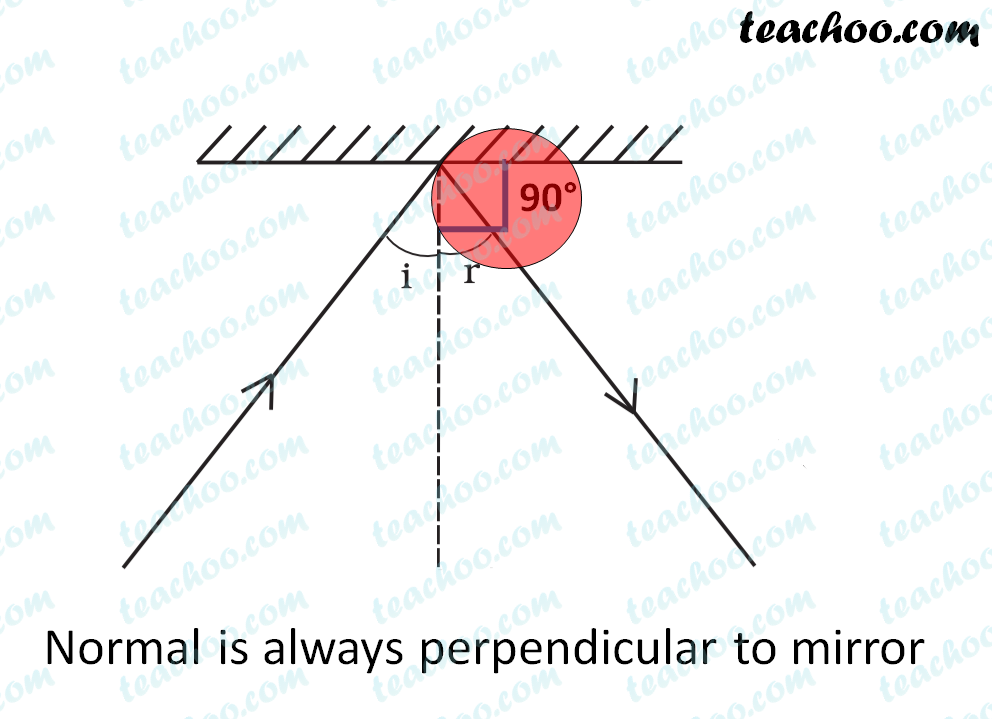## Show how the word would appear in the mirror.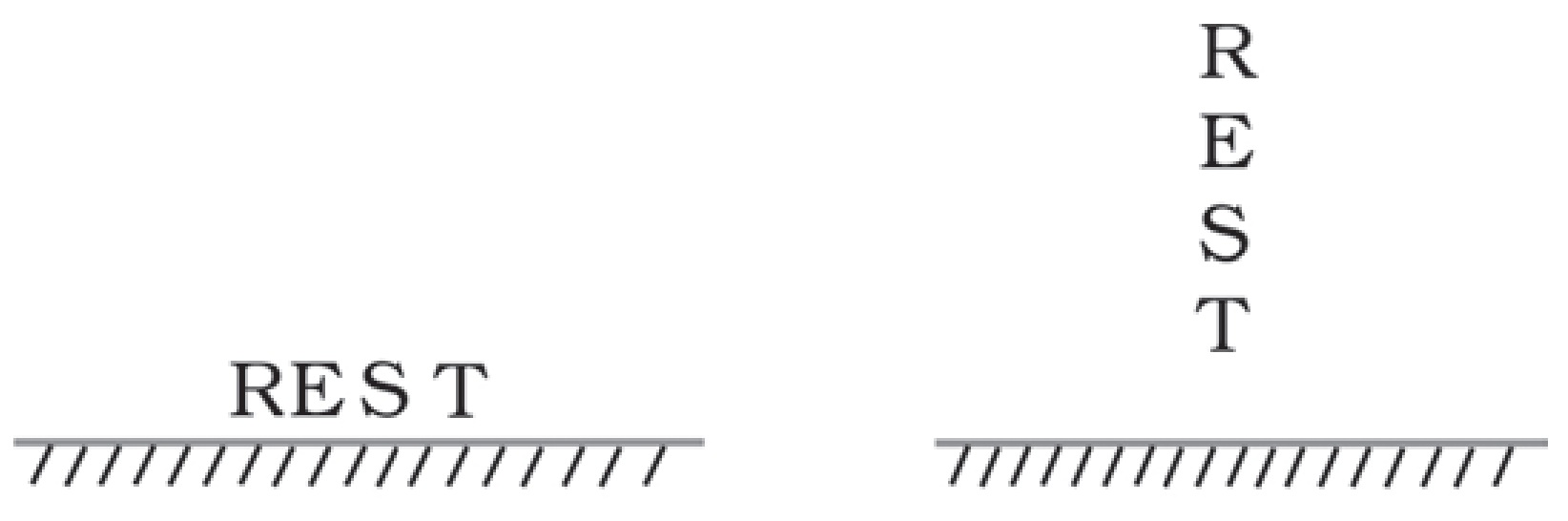In the image formed by plane mirror, Image is

• Laterally inverted
• Distance of image from mirror is same as distance of object from mirror

So, our images look like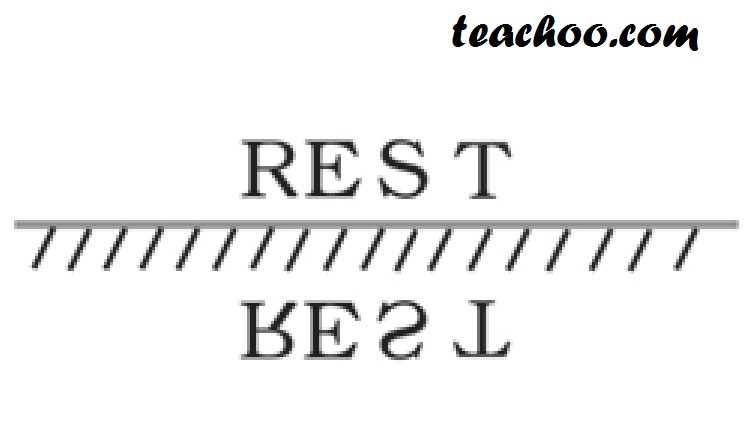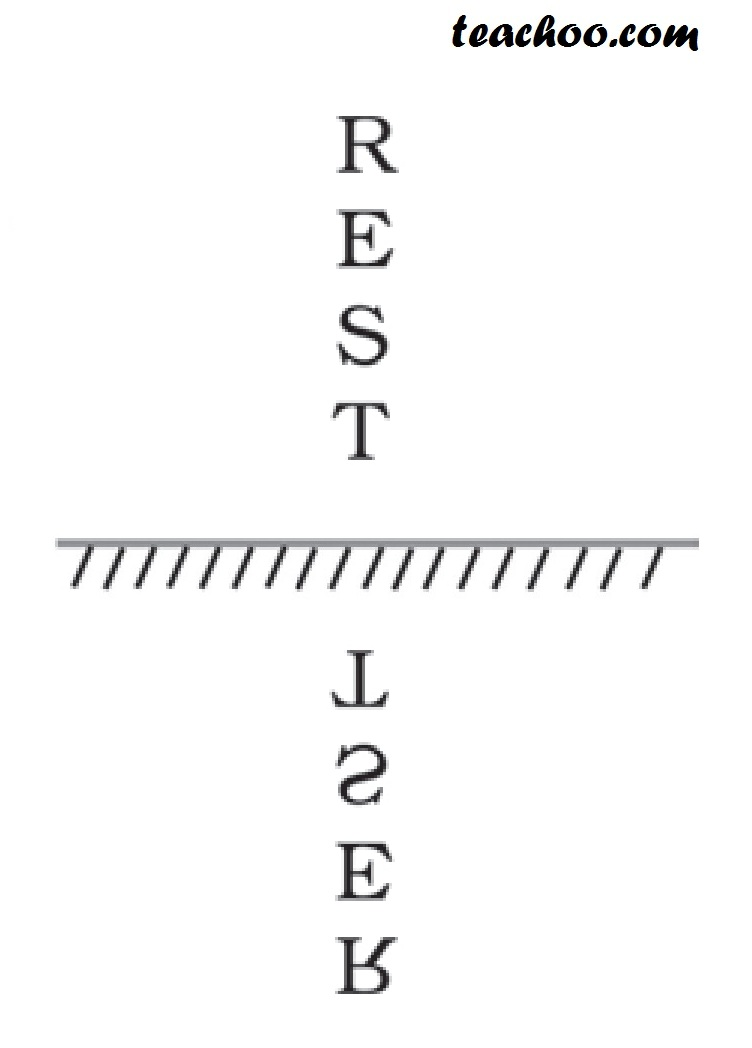1. Class 8
2. Chapter 16 Class 8 - Light
3. Teachoo Questions

Teachoo Questions

Class 8
Chapter 16 Class 8 - Light